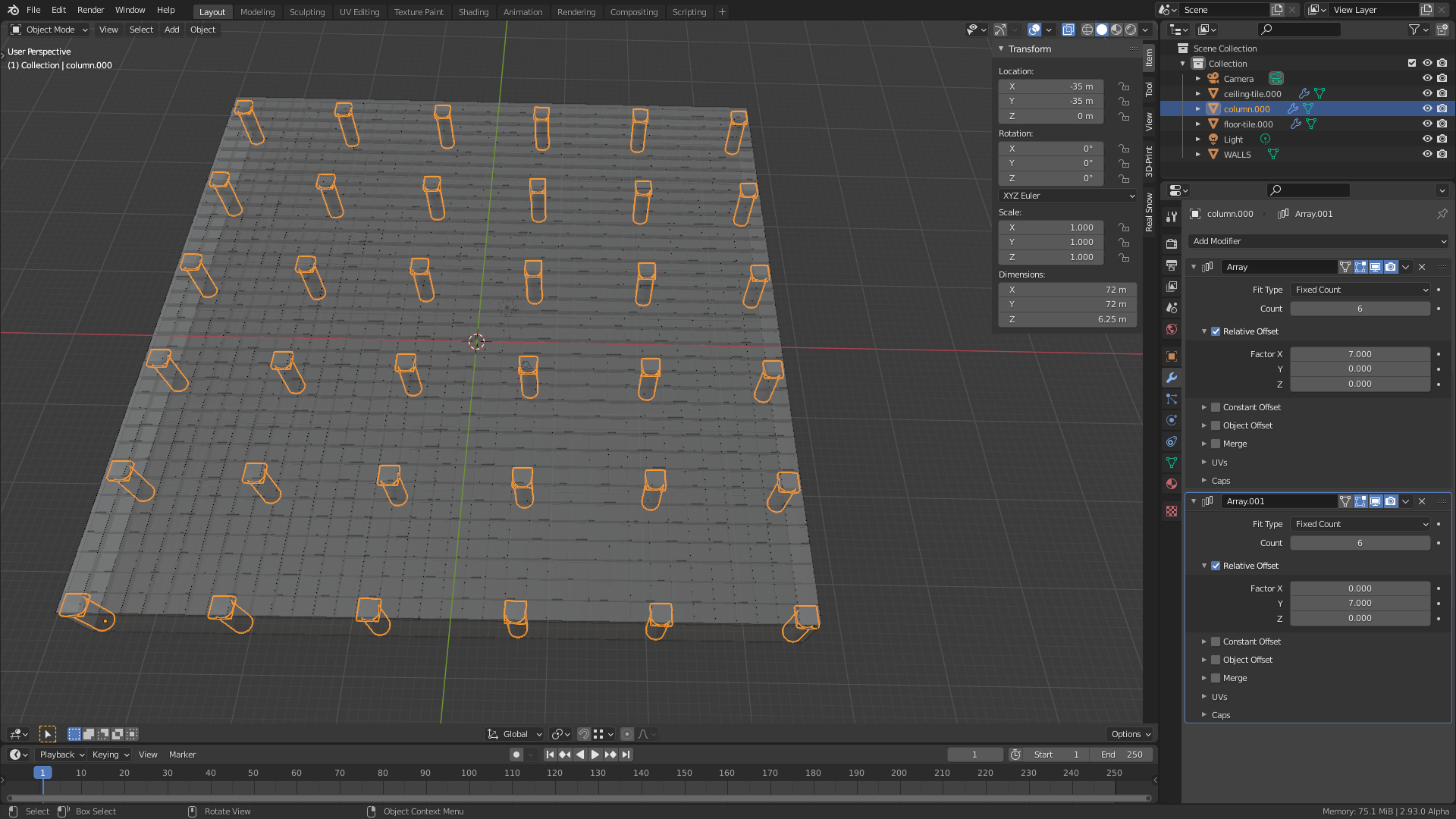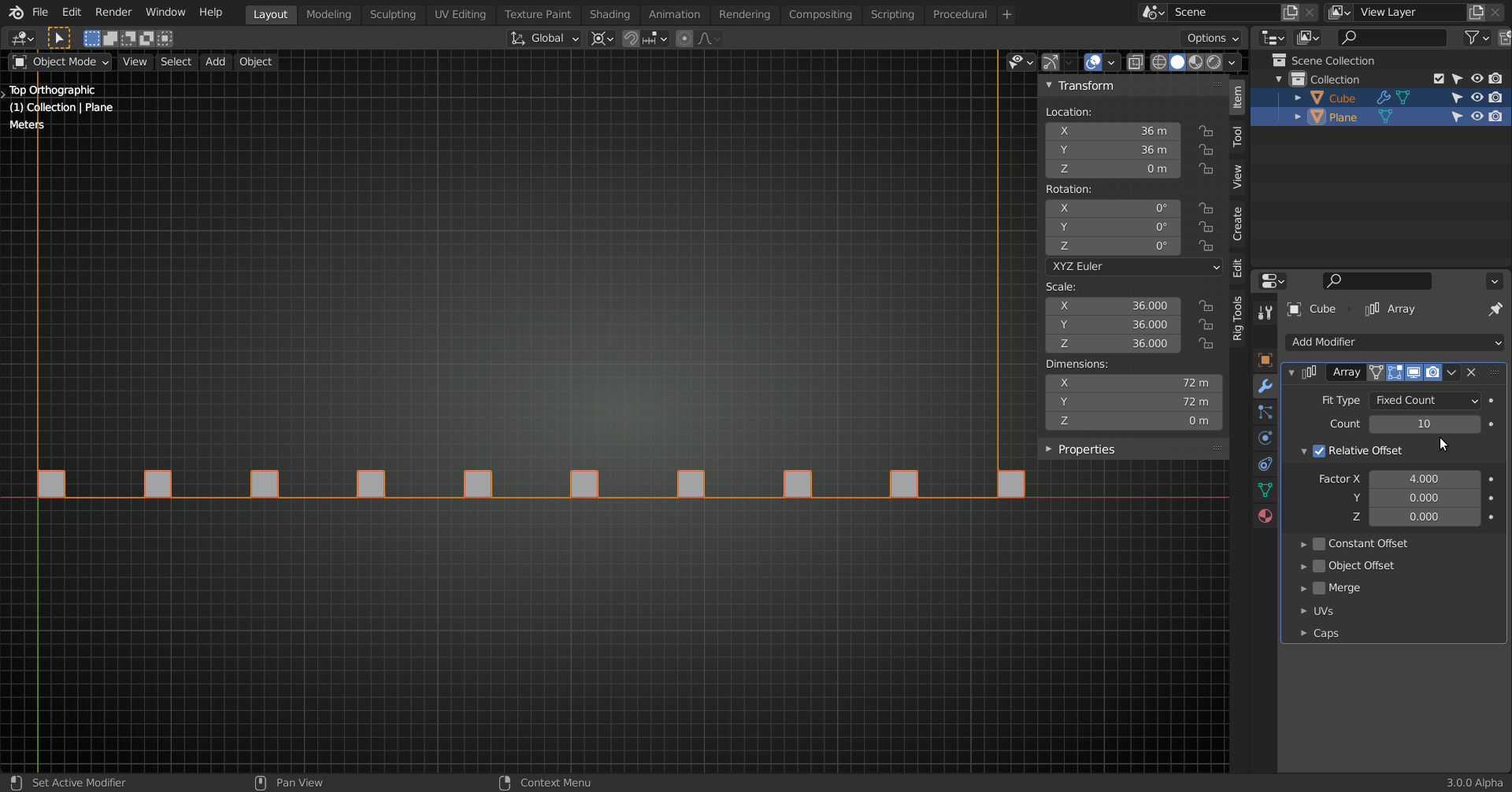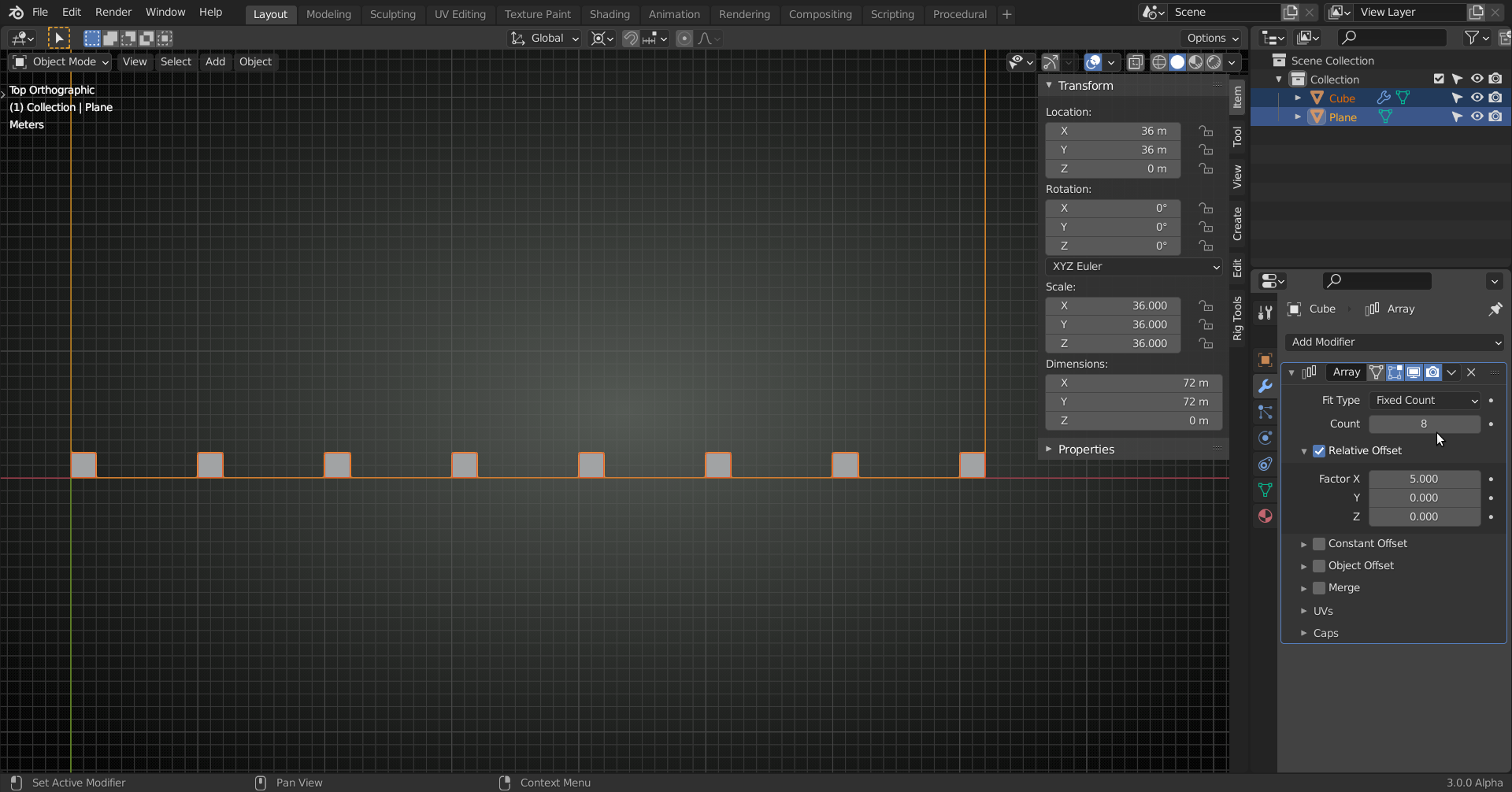## How many squared Columns can be arranged on a squared Parking Level using the "Array Modifier" without any Intersections?

Not an easy answer for me but maybe for an excellent mathematician likespikeyxxx. For the sake of simplicity, we assume that the distance between one column and the next one is a whole number multiple of the column's "Bounding Box" dimension in the direction of duplication.

So here're my reflections:

We use the "Array Modifier" with its default settings "Fixed Count" and "Relative Offset" (X=1, Y=0, Z=0) so that we get a whole number of duplicates tightly packed along their aligned  "Local X Axes" where each duplicate's "Bounding Box" touches the "Bounding Box" of its direct neighbour. With our 72 times 72 meters parking level and 2 times 2 meters columns, 36 columns would fit into the garage with the "Array Modifier's" default settings. But this construction wouldn't be useful 😉😁!

We could set the "Relative Offset" to a higher value so that we get a whole number multiple of our object's "Bounding Box" extension in "Local X". This offset can be a "Decimal Fraction" but for the sake of simplicity mentioned at the beginning, we limit ourselves in the following to whole numbers.

What combinations are possible in general?

Two criterias must be fulfilled after subtracting one time the duplicated object's "Bounding Box" extension in the direction of duplication (default: "Local X"):

1) The result of this first subtraction must be divisible by a whole number so that you get again a whole number.

2) This division result must be a whole number which is at the same time a whole number multiple the duplicated object's "Bounding Box" extension in the direction of duplication (still "Local X" in our example 😉)

Unless you're good in mental math and have a lot of time (depending on the specific dimensions of your scene 😉), you would need a programm for automated calculations, maybe one you've developed yourself 😉. So, Kenttheluthier, don't worry, you're not alone with your headache when figuring out settings for the "Array Modifier" that make the columns fit the dimensions of the parking level😉!

Finally, this is the specific parking level at the end of this lesson:• For the answer, lets first look at a related, but simpler problem:

we will try and divide a side of 36 (72 divided by the length of the default cube) in equal parts. To do this, we look at the prime factorization of 72:

2x2x3x3. Consider all possible combinations of those primes and their product (including the 'empty' product, where none of them is being multiplied, resulting in 1).

We get: 1, 2, 3, 4, 6, 9, 12, 18 and 36. These are actually pairs of whole number solutions to the equation : A x B = 36.

So we have 9 possible divisions, for instance:But the two corners aren't occupied. If we add one more cube:that will lie exactly outside of our garage.

If our Plane were one cube larger, our problem would be solved. And this already gives us the solution to the original problem:

We divide 72 -2 = 70 (side length minus one cube )  by 2 (cube length) and get 35.

Then look at the prime factorization: 35 = 5 x 7. This yields two possibilities:

Array count of 6 (5 plus 1!), with a Relative Offset of 7 and an Array count of 8 (7 plus 1) with a Relative Offset of 5.•spikeyxxx This is a very elegant method to find all possible solutions, 😀! Fun fact: I also supposed that the solution could maybe have to do with prime numbers 🧮. But this was just my intuition since these numbers seem to play an important role in mathematics and are mentioned in various places.

One more question: What would the calculations look like if we allowed decimal fractions as offset?

• What would the calculations look like if we allowed decimal fractions as offset?

Very simple!

Subtract one cube length from the garage side length. then divide the garage length by the column length and decide how many columns you want. Divide the reduced garage length by the number of columns minus 1 to get the Relative Offset:• I also supposed that the solution could maybe have to do with prime numbers

Yes, every time, you get a problem with whole numbers, you should probably look at prime factorization.

Unless you are talking about really large numbers; in that case it might be really hard (even with computers) to find that (always unique!) prime factorization. In fact, that is why SHA cryptography works, They multiply two large primes and make the result known (public key). But to encrypt and decrypt, you will need those primes and unless you know at least one of them, you are in deep trouble ;)

•spikeyxxx Isn't there a kind of competition finding the largest known prime number? I don't know where they are currently, who/which team did it and what supercomputer was used in order to calculate it.

•duerer I know there are always people looking to find larger and larger primes, maybe there is a competition, doesn't seem unlikely to me ;)

The way they go about finding them is interesting; they take a large number and then do some tests on it. As soon as it fails one of the tests, it is definitely not a prime. So the more tests it passes, the more likely it becomes, that that number is  a prime.

I do not know, how they decide that it is indeed a prime....maybe, they try to use it to encrypt and decrypt some message and see if that works...if it is not a prime, they will not be able to decrypt it with the SHA algorithm (if I am not mistaken....). So if the decryption works, it must be a prime number.

• In the last solution, I wasn't very consequent and left out the (practically useless) trivial solutions involving 1 and 35, that would give an Array Count of 36 (35 plus 1) with Relative Offset of 1 and  an Array Count of 2 (1 plus 1) with a Relative Offset of 35.

• Woah. I definitely don't feel bad about not knowing how to explain this math in the video now 😅